## Some basic concepts of chemistry Quiz-39

IIT JEE exam which consists of JEE Main and JEE Advanced is one of the most important entrance exams for engineering aspirants. The exam is held for candidates who are aspiring to pursue a career in the field of engineering and technical studies. Chemistry is important because everything you do is chemistry! Even your body is made of chemicals. Chemical reactions occur when you breathe, eat, or just sit there reading. All matter is made of chemicals, so the importance of chemistry is that it's the study of everything..

Q1.How many moles of magnesium phosphate, Mg_2 (PO_4 )_2 will contain 0.25 mole of oxygen atoms?
•  0.02
•  3.125×10^(-2)
•  1.25×10^(-2)
•  2.5×10^(-2)
Solution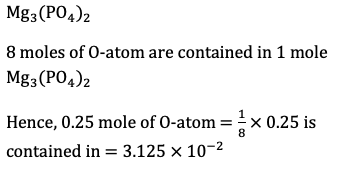Q2.Which is the volatile compound burning with green flame?
•  (C_2 H_5 )_3 B
•  (C_2 H_5 )_3 BO_3
•  Ba(NO_3 )_2
•  BaCl_2
Solution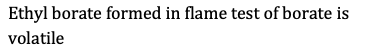Q3. In an experiment, 20 mL of 0.1 M solution of a metallic salt reacted exactly with 25 mL of 0.1 M solution of sodium sulphite. In the reaction, SO_3^(2-) is oxidised to SO_4^(2-). If the original oxidation number of the metal in the salt was 3, what would be the new oxidation number of the metal?
•  0
•  1
•  2
•  4
Solution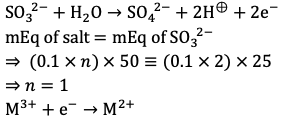Q4. A boy drinks 500 L of 9% glucose solution. The number of glucose molecules he has consumed is [mol. Wt. of glucose = 180]
•  0.5×10^23
•  1.0×10^23
•  1.5×10^23
•  2.0×10^23
Solution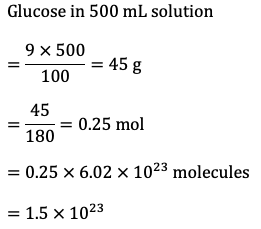Q5. Atomic weight of barium is 137.34. The equivalent weight of barium in BaCrO_4 used as an oxidizing agent in acid medium is
•  137.34
•  45.78
•  114.45
•  68.67
Solution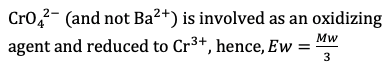Q6. How many grams of phosphoric acid would be needed to neutralise 100 g of magnesium hydroxide? (The molecular weights are: H_3 PO_4=98 and Mg(OH)_2= 58.3)
•  66.7 g
•  252 g
• 112 g
•  168 g
Solution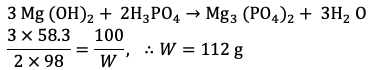Q7. Dissolving 120 g of urea (60 g mol^(-1) ) in 1000 g of water gave a solution of density 1.15 g/mL.The molarity of solution is
•  1.78 M
•  2.00 M
•  2.05 M
•  IV, III
Solution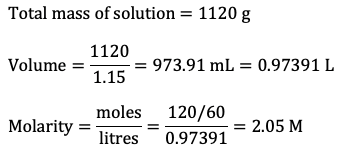Q8.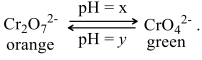This change is based on change in pH. Probable values of x and y can be
•  8, 6
•  8, 10
•  4, 6
•  Change is independent of pH
Solution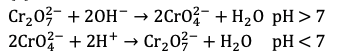Q9. 2 L of air formed 1915 mL of ozonised air when passed through Brodio’s apparatus. The volume of ozone formed is
•  85 mL
•  170 mL
•  225 mL
•  42.5 mL
Solution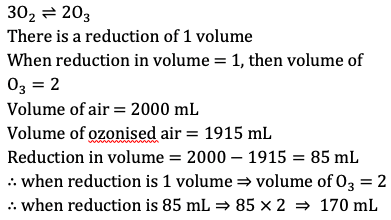Q10. Aqueous solution contains ZnCl_2,CdCl_2 and CuCl_2. On passing H_2 S gas, there is precipitation of…. as sulphide
•  Zn^(2+),Cd^(2+)
•  Cu^(2+),Cd^(2+)
•  Zn^(2+),Cu^(2+)
• n^(2+),Cu^(2+),Cd^(2+)
Solution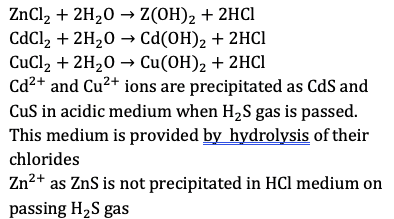#### Written by: AUTHORNAME

AUTHORDESCRIPTION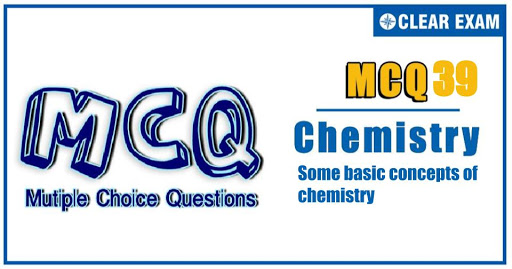## Want to know more

Please fill in the details below:

## Latest NEET Articles\$type=three\$c=3\$author=hide\$comment=hide\$rm=hide\$date=hide\$snippet=hide

Name

ltr
item
BEST NEET COACHING CENTER | BEST IIT JEE COACHING INSTITUTE | BEST NEET & IIT JEE COACHING: SOME BASIC CONCEPTS OF CHEMISTRY Quiz-39
SOME BASIC CONCEPTS OF CHEMISTRY Quiz-39